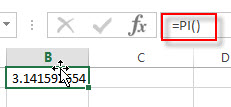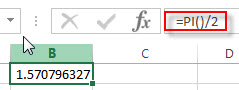# Excel PI Function

This post will guide you how to use Excel PI function with syntax and examples in Microsoft excel.

### Description

The Excel PI function returns the value of mathematical constant PI. The returned value is 3.14159265358979 and ti will accurate to 15 digits.

The PI function is a build-in function in Microsoft Excel and it is categorized as a Math and Trigonometry Function.

The PI function is available in Excel 2016, Excel 2013, Excel 2010, Excel 2007, Excel 2003, Excel XP, Excel 2000, Excel 2011 for Mac.

### Syntax

The syntax of the PI function is as below:

`=PI()`

The PI function has no any arguments.

### Excel PI Function Examples

The below examples will show you how to use Excel PI Function to return the value of PI.

1# get the value of PI, enter the following formula in Cell B1.

=PI()2# get the value of PI divided by 2, enter the following formula in Cell B2.

=PI()/2